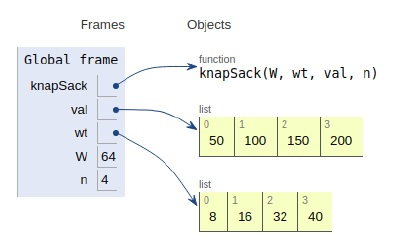# Python Program for 0-1 Knapsack Problem

Problem statement − We are given weights and values of n items, we need to put these items in a bag of capacity W up to the maximum capacity w. We need to carry a maximum number of items and return its value.

Now let’s observe the solution in the implementation below −

# Brute-force approach

## Example

Live Demo

#Returns the maximum value that can be stored by the bag
def knapSack(W, wt, val, n):
# initial conditions
if n == 0 or W == 0 :
return 0
# If weight is higher than capacity then it is not included
if (wt[n-1] > W):
return knapSack(W, wt, val, n-1)
# return either nth item being included or not
else:
return max(val[n-1] + knapSack(W-wt[n-1], wt, val, n-1),
knapSack(W, wt, val, n-1))
# To test above function
val = [50,100,150,200]
wt = [8,16,32,40]
W = 64
n = len(val)
print (knapSack(W, wt, val, n))

## Output

350

#dynamic approach

## Example

Live Demo

# a dynamic approach
# Returns the maximum value that can be stored by the bag
def knapSack(W, wt, val, n):
K = [[0 for x in range(W + 1)] for x in range(n + 1)]
#Table in bottom up manner
for i in range(n + 1):
for w in range(W + 1):
if i == 0 or w == 0:
K[i][w] = 0
elif wt[i-1] <= w:
K[i][w] = max(val[i-1] + K[i-1][w-wt[i-1]], K[i-1][w])
else:
K[i][w] = K[i-1][w]
return K[n][W]
#Main
val = [50,100,150,200]
wt = [8,16,32,40]
W = 64
n = len(val)
print(knapSack(W, wt, val, n))

## Output

350All the variables are declared in the local scope and their references are seen in the figure above.

## Conclusion

In this article, we have learned about how we can make a Python Program for 0-1 Knapsack Problem